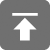# 工学1号馆

home

## C语言结构体中的函数指针

Wu Yudong    June 27, 2015     C   453

### 引言

int * intptr;     // 声明一个指向整型值的指针
int intval = 5 ;  // 定义一个整型变量
intptr = & intval ; // intptr现在包含intval的地址

### 函数指针

Return Type  * function pointer’s variable name ) ( parameters

int (*func)(int a , int b ) ;

typedef int (*func)(int a , int b ) ;

### 结构体中的函数指针

typedef int (*Operation)(int a , int b );

typedef  struct _str {
int  result ; // 用来存储结果
Operation  opt; // 函数指针

} STR;

//a和b相加
int Add (int a, int b){
return a + b ;
}
//a和b相乘
int Multi (int a, int b){
return a * b ;
}

int main (int argc , char **argv){
STR str_obj;
str_obj. result = str_obj.opt(5,3);
printf (" the result is %d\n", str_obj.result );
str_obj.opt= Multi;    //函数指针变量指向Multi函数
str_obj. result = str_obj.opt(5,3);
printf (" the result is %d\n", str_obj.result );
return 0 ;
}

the result is 8

the result is 15

#include<stdio.h>

typedef int (*Operation)(int a, int b);
typedef struct _str {
int result ; // to sotre the resut
Operation  opt; // funtion pointer
} STR;

//a和b相加
int Add (int a, int b){
return a + b ;
}

//a和b相乘
int Multi (int a, int b){
return a * b ;
}

int main (int argc , char **argv){
STR str_obj;
str_obj. result = str_obj.opt(5,3);
printf ("the result is %d\n", str_obj.result );
str_obj.opt= Multi;    //函数指针变量指向Multi函数
str_obj. result = str_obj.opt(5,3);
printf ("the result is %d\n", str_obj.result );
return 0 ;
}